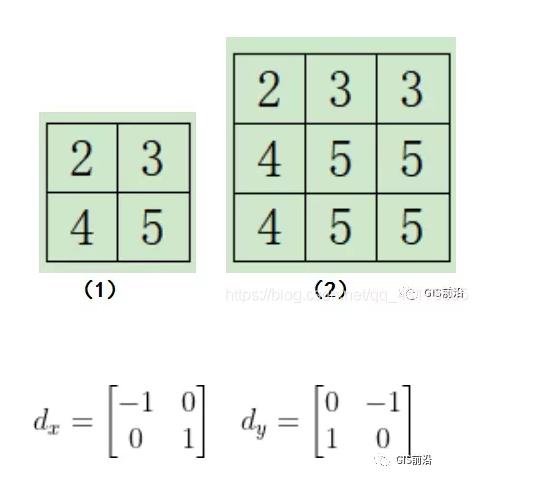﻿ 基于Java实现空间滤波完整代码_java_脚本之家
java# 基于Java实现空间滤波完整代码

## 图像需要增强的原因

(1) 中值滤波

PART/014 4 3 7 6 8 8

4 4 3 7 6 8 8

2 2 15 8 9 9 9

5 5 8 9 13 10 10

7 7 9 12 15 11 11

8 8 11 10 14 13 13

8 8 11 10 14 13 13```package NB;

import java.util.ArrayList;

import java.util.Arrays;

import java.util.Scanner;

public class zzlb {

public static void main(String[] args) {

Scanner s=new Scanner(System.in);

System.out.println("请输入矩阵的行数：");

int x=s.nextInt();//获取键盘输入的数字

System.out.println("请输入矩阵的列数：");

int y=s.nextInt();//获取键盘输入的数字

int[][]b=new int[x][y];//创建一个二维数组

int[]p=new int;//创建一个可以存放9个元素的一维数组来获取#3*3窗口的像元值

System.out.println("请输入"+x+"*"+y+"的矩阵：");

for (int i = 0; i <y; i++) {

for (int j = 0; j < x; j++) {

b[i][j]=s.nextInt();//将键盘输入的矩阵存放到二维数组里面

}

}

System.out.print("运算结果如下：\n");

for (int i = 1; i <y-1; i++) {

for (int j = 1; j < x-1; j++) {  //锁定到中心像元的位置，从（1，1）开始

int g=0;

for (int k =i-1; k <=i+1 ; k++) {

for (int l =j-1; l <=j+1 ; l++) {

p[g++]=b[k][l]; //将3*3矩阵窗口存储到一维数组中

}

}

for (int k = 0; k <p.length-1; k++) {

for (int l = 0; l < p.length-1-k; l++) {

if(p[l]>p[l+1]) {

int temp = p[l];

p[l]=p[l+1];

p[l+1]=temp;//本次采用冒泡排序法对3*3窗口内的像元从小到大排序

}

}

}

System.out.print(p+" ");//输出每个3*3模板的中心值，下标都是4

}

System.out.println("\n");//输出完一行就换行

}

}
}```

（2）罗伯特锐化

PART/02F=|aidxi|+|aidyi|，其中a是矩阵中的2*2个元素，i是第i个元素，i<=4,计算结果放在左上角像元，代替之前的灰度值，但是右下角的像元模板范围内没有其他像元了，无法进行计算，为此使用罗伯特锐化方法都要在原始矩阵的最下边和最右边添加一行和一列，值都和最临近的像元灰度值一样。为了直白了断，小编直接上例子吧，比如有某个矩阵，如下图（1）所示，我要用上面两个模板进行罗伯特锐化，首先得在右边和下边分别添加一行和一列，如下图（2）所示：2 2 10 10 10 10

2 2 10 10 10 10

2 2 10 10 10 10

2 2 2 2 2 2

2 2 2 2 2 2

2 2 2 2 2 2

```package NB;

import java.util.Scanner;

public class robet {
public static void main(String[] args) {

Scanner s1 = new Scanner(System.in);

System.out.println("请输入矩阵的行数：");

int x = s1.nextInt();//获取键盘输入的数字

System.out.println("请输入矩阵的列数：");

int y = s1.nextInt();//获取键盘输入的数字

int[][] b = new int[x][y];//创建一个二维数组

System.out.println("请输入" + x + "*" + y + "的矩阵：");

for (int i = 0; i < y; i++) {

for (int j = 0; j < x; j++) {

b[i][j] = s1.nextInt();

}///将键盘输入的矩阵存放到二维数组里面

}

for (int i =0; i <y-1; i++) {

for (int j = 0; j <x-1; j++) {

b[i][j]=Math.abs(b[i][j]-b[i+1][j+1])+Math.abs(b[i+1][j]-b[i][j+1]);

System.out.print(b[i][j]+" ");

}//对两个模板进行图像卷积运算后取绝对值相加，并且把结果放到2*2窗口的左上方

System.out.println("\n");//输出完一行就换行

}

}
}```